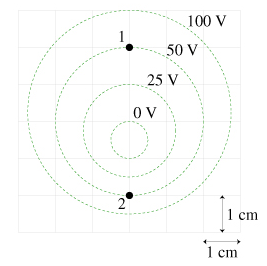# Problem: a.) Determine the magnitude of the electric field at point 1 in the figure.b.) Determine the magnitude of the electric field at point 2 in the figure.(Note the grid in the background.)

###### FREE Expert Solution

Electric field and electric potential are related by

$\overline{){\mathbf{E}}{\mathbf{=}}{\mathbf{-}}\frac{\mathbf{∆}\mathbf{V}}{\mathbf{∆}\mathbf{x}}}$

99% (341 ratings)###### Problem Detailsa.) Determine the magnitude of the electric field at point 1 in the figure.

b.) Determine the magnitude of the electric field at point 2 in the figure.

(Note the grid in the background.)